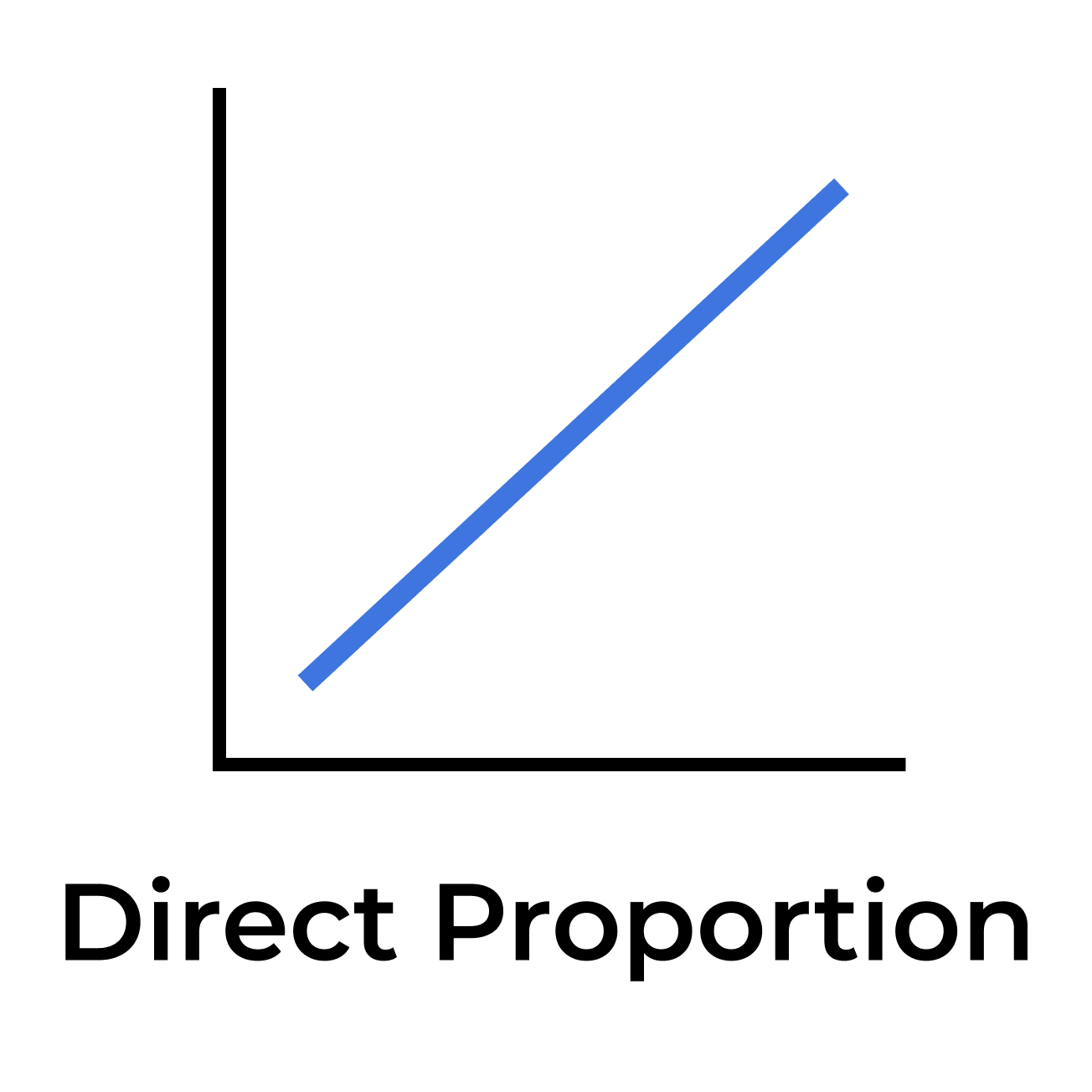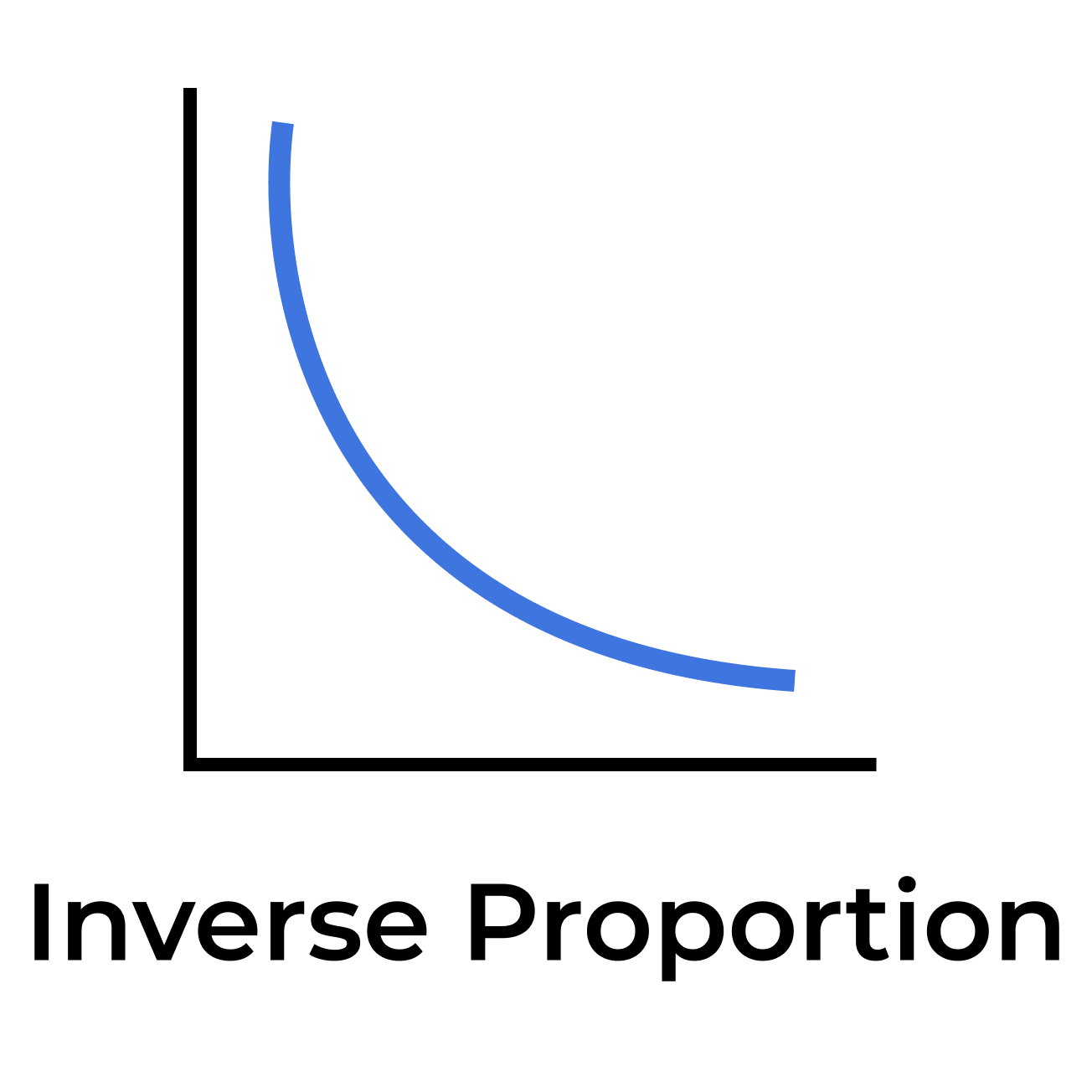Uh Oh! It seems you’re using an Ad blocker!

We always struggled to serve you with the best online calculations, thus, there's a humble request to either disable the AD blocker or go with premium plans to use the AD-Free version for calculators.

Or# Proportion Calculator

Note: Enter 3 numbers and unknown variable (x) or any other letter into the given fields.

=

Table of Content

Get the Widget!

Add this calculator to your site and lets users to perform easy calculations.

Feedback

How easy was it to use our calculator? Did you face any problem, tell us!

Proportion calculator solves two ratios to determine the value of the missing variable x. Compare two fractions with an equivalent sign and get complete solution in one click.

## What Are Proportions?

“Proportion is said to be a mathematical comparison between two ratios (fractions)”

Symbol: “$$::$$” or “$$=$$”## Proportion Types:

There are two kinds that includes:

### Direct Proportion:In this type, increase/decrease in one quantity causes an increase/decrease in the second quantity.

Mathematically:

a ∝ b

or, a/b = k

Where, k = A positive integer and “a” and “b” are directly proportional relationship to each other.

### Inverse Proportion:In this case, if one quantity increases, the other decreases. Similarly if the first quantity decreases, the second one increases.

Mathematically:

a ∝ 1/b

Where, the relation between “a” and “b” are inverse to each other.

## How To Solve Proportions Manually (Step-by-Step):

If you want to know the proportion with 2 variables from the proportion equation, then

• Simply put the equal sign between them
• Find the missing value by cross multiplication

### Example:

The equation is given as $$\dfrac {8}{x} = \dfrac {6}{4}$$, solve for the unknown $$x$$?

#### Solution:

By Cross Multiplication:

Try the simple proportions calculator to solve each proportion problems with fractions. As the equation is given as follows:

$$\frac {8}{x} = \frac {6}{4}$$

The cross-multiplication method is a common technique for solving proportions. So, by this we get:

$$6x = 8 \times 4$$

$$x = 8 \times \frac {4}{6}$$

$$x = \frac {32}{6}$$

$$x = 5.33$$

The Equation is Equal if,

8 / 6 = 1.33

So, it is true that,

x / 4 = 1.33

x = 1.33 × 4

x = 5.33

## References:

From the source of Wikipedia : Understanding of direct & inverse

From the site of mathisfun : Definition.